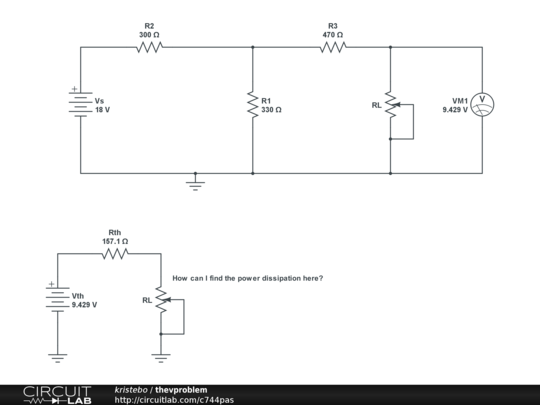# How can I find maximum power transfer using Thevenin equivalentAs I understand it, should maximum power transfer be when $$R_L=R_{th}$$ then $$P_L= P_{L(max)}$$

So that this should do the trick:

$$P_{L}=\frac{V_{th} ^2}{R_{th}}$$

But it seems that I am doing something wrong.

$P_{L,max} = \dfrac{V^2_{th}}{4R_{th}}$